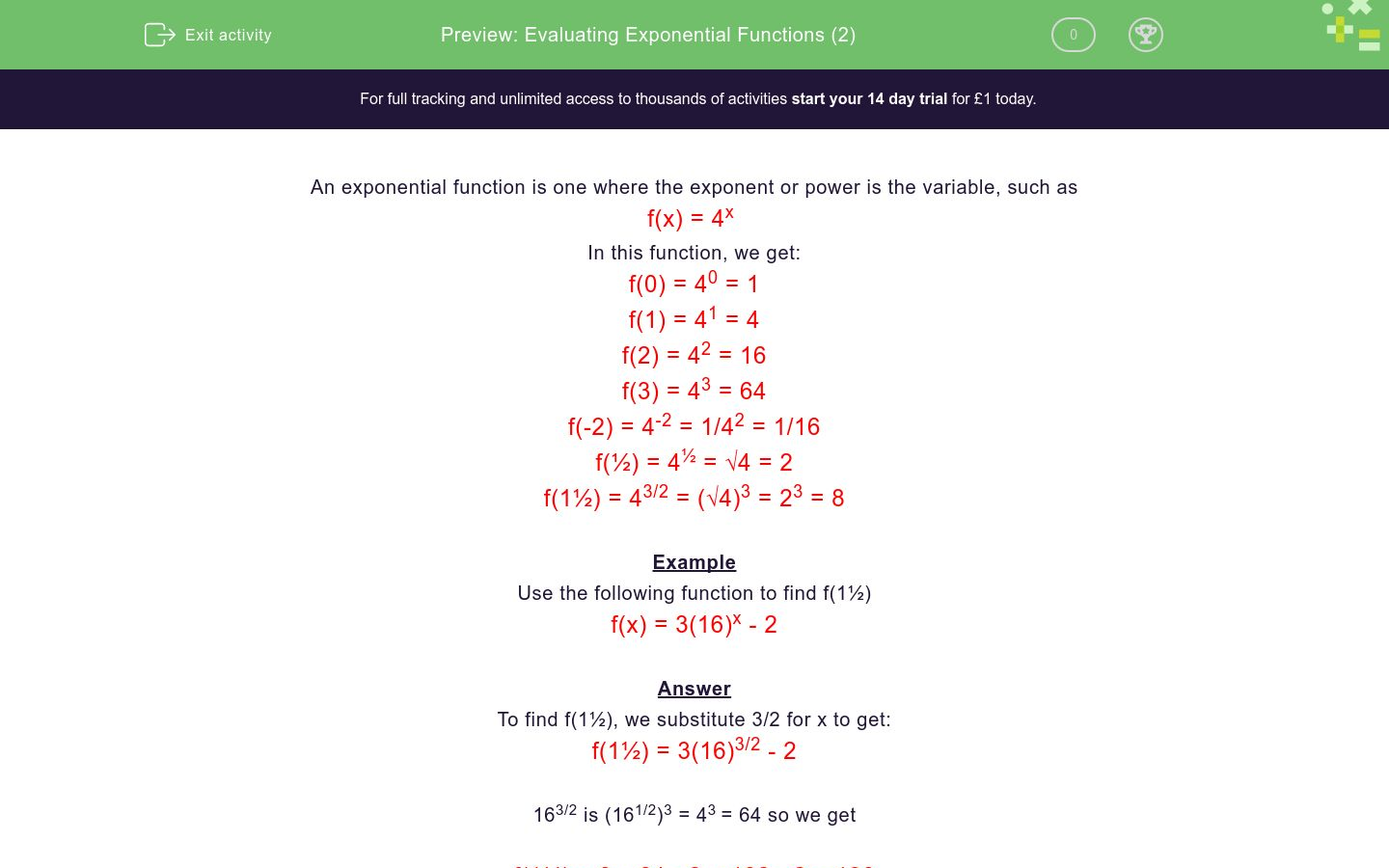# Evaluating Exponential Functions (2)

In this worksheet, students substitute numerical values (including negative numbers and fractions) into exponential functions.Key stage:  KS 4

Curriculum topic:  Algebra

Difficulty level:### QUESTION 1 of 10

An exponential function is one where the exponent or power is the variable, such as

f(x) = 4x

In this function, we get:

f(0) = 40 = 1

f(1) = 41 = 4

f(2) = 42 = 16

f(3) = 43 = 64

f(-2) = 4-2 = 1/42 = 1/16

f(½) = 4½ = √4 = 2

f(1½) = 43/2 = (√4)3 = 23 = 8

Example

Use the following function to find f(1½)

f(x) = 3(16)x - 2

To find f(1½), we substitute 3/2 for x to get:

f(1½) = 3(16)3/2 - 2

163/2 is (161/2)3 = 43 = 64 so we get

f(1½) = 3 x 64 - 2 = 192 - 2 = 190

f(1½) = 190

Use the following function to find f(½)

f(x) = 4x

Use the following function to find f(½)

f(x) = 9x + 3

Use the following function to find f(-2)

f(x) = (2)x + 7

Use the following function to find f(-2)

f(x) = 2(4)x + 7

Use the following function to find f(-4)

f(x) = 5x + 1

Use the following function to find f(0)

f(x) = 88x + 49

Use the following function to find f(½)

f(x) = 7(25)x + 4

Use the following function to find f(3/2)

f(x) = 25x - 44

Use the following function to find f(-½)

f(x) = 16x + 44

Use the following function to find f(½)

f(x) = (¼)x - ½

• Question 1

Use the following function to find f(½)

f(x) = 4x

2
EDDIE SAYS
4½ = √4
• Question 2

Use the following function to find f(½)

f(x) = 9x + 3

6
EDDIE SAYS
9½ + 3 = √9 + 3
• Question 3

Use the following function to find f(-2)

f(x) = (2)x + 7

7.25
EDDIE SAYS
2-2 + 7 = 1 ÷ 4 + 7
• Question 4

Use the following function to find f(-2)

f(x) = 2(4)x + 7

7.125
EDDIE SAYS
2 × 4-2 + 7 = 2 × (1/16) + 7
• Question 5

Use the following function to find f(-4)

f(x) = 5x + 1

1.0016
EDDIE SAYS
5-4 + 1 = 1/625 + 1
• Question 6

Use the following function to find f(0)

f(x) = 88x + 49

50
EDDIE SAYS
880 + 49 = 1 + 49
• Question 7

Use the following function to find f(½)

f(x) = 7(25)x + 4

39
EDDIE SAYS
7 × 25½ + 4 = 7 × √25 + 4
• Question 8

Use the following function to find f(3/2)

f(x) = 25x - 44

81
EDDIE SAYS
253/2 - 44 = (√25)3 - 44 = 125 - 44
• Question 9

Use the following function to find f(-½)

f(x) = 16x + 44

44.25
EDDIE SAYS
16 + 44 = 1/√16 + 44
• Question 10

Use the following function to find f(½)

f(x) = (¼)x - ½

0
EDDIE SAYS
¼½ - ½ = √¼ - ½ = ½ - ½
---- OR ----

Sign up for a £1 trial so you can track and measure your child's progress on this activity.

### What is EdPlace?

We're your National Curriculum aligned online education content provider helping each child succeed in English, maths and science from year 1 to GCSE. With an EdPlace account you’ll be able to track and measure progress, helping each child achieve their best. We build confidence and attainment by personalising each child’s learning at a level that suits them.

Start your £1 trial